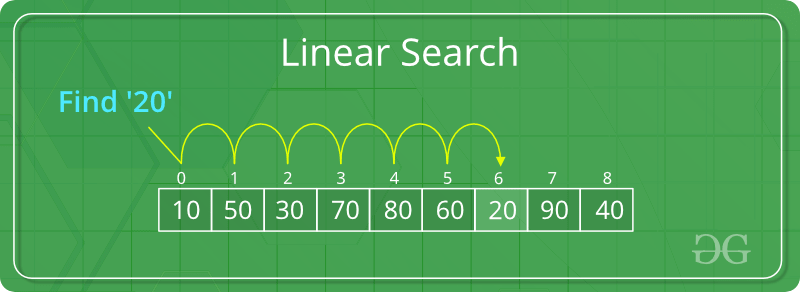# Python | Linear search on list or tuples

• Last Updated : 16 Jan, 2019

Let’s see a basic linear search operation on Python list and tuples.

A simple approach is to do linear search, i.e

Attention geek! Strengthen your foundations with the Python Programming Foundation Course and learn the basics.

To begin with, your interview preparations Enhance your Data Structures concepts with the Python DS Course. And to begin with your Machine Learning Journey, join the Machine Learning - Basic Level Course

• Start from the leftmost element of list and one by one compare x with each element of the list.
• If x matches with an element, return True.
• If x doesn’t match with any of elements, return False.Example #1: Linear Search on Lists

 `# Search function with parameter list name``# and the value to be searched``def` `search(``list``,n):`` ` `    ``for` `i ``in` `range``(``len``(``list``)):``        ``if` `list``[i] ``=``=` `n:``            ``return` `True``    ``return` `False`` ` `# list which contains both string and numbers.``list` `=` `[``1``, ``2``, ``'sachin'``, ``4``,``'Geeks'``, ``6``]`` ` `# Driver Code``n ``=` `'Geeks'`` ` `if` `search(``list``, n):``    ``print``(``"Found"``)``else``:``    ``print``(``"Not Found"``)`
Output:
```Found
```

Note that list are mutable but tuples are not.

Example #2: Linear Search in Tuple

 `# Search function with parameter list name``# and the value to be searched``def` `search(``Tuple``, n):`` ` `    ``for` `i ``in` `range``(``len``(``Tuple``)):``        ``if` `Tuple``[i] ``=``=` `n:``            ``return` `True``    ``return` `False`` ` `# list which contains both string and numbers.``Tuple``=` `(``1``, ``2``, ``'sachin'``, ``4``, ``'Geeks'``, ``6``)`` ` ` ` `# Driver Code``n ``=` `'Geeks'`` ` `if` `search(``Tuple``, n):``    ``print``(``"Found"``)``else``:``    ``print``(``"Not Found"``)`
Output:
```Found
```

My Personal Notes arrow_drop_up